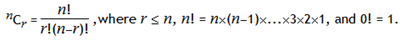# Project Euler – Problem 53 Solution

#### Problem

There are exactly ten ways of selecting three from five, 12345:

123, 124, 125, 134, 135, 145, 234, 235, 245, and 345

In combinatorics, we use the notation, 5C3 = 10.

In general,It is not until n = 23, that a value exceeds one-million: 23C10 = 1144066.

How many, not necessarily distinct, values of  nCr, for 1 <= n <= 100, are greater than one-million?

#### Solution

```let factorial n = if (n = 0I) then 1I else [1I..n] |> List.reduce (*)

let C n r = if r <= n then (factorial n) / ((factorial r) * (factorial (n - r))) else 0I